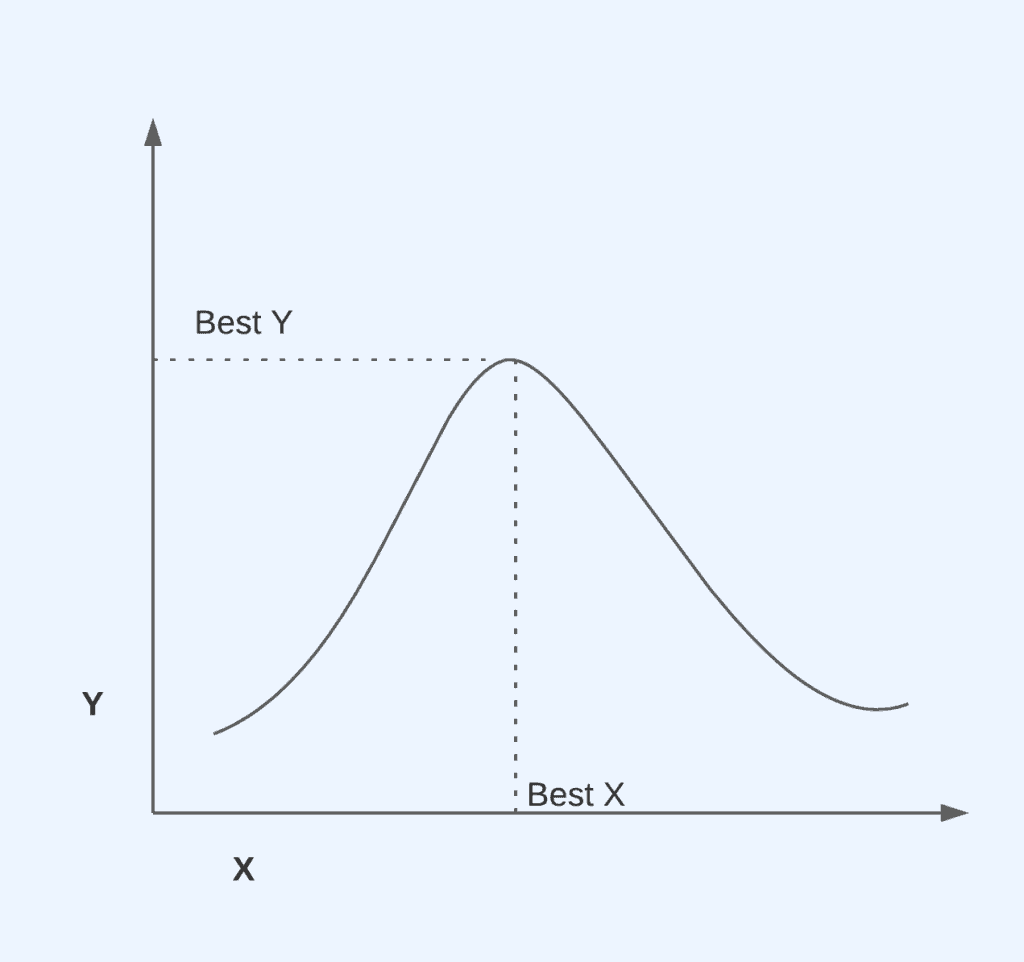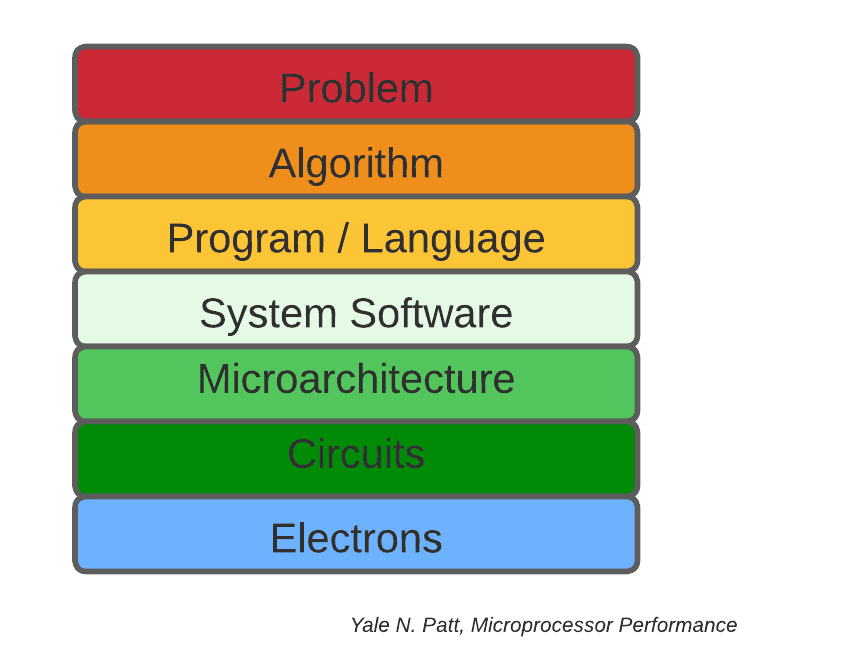## 1. Overview

In this tutorial, we’ll start with the general definition of optimization. Then, we’ll narrow it down to subject programming optimization. It is one of the essential concepts in computing science. It wouldn’t be exaggerating to say that without optimization, computing science wouldn’t come so far today.

## 2. Optimization Definition

So, if we start with its general definition, it is about finding minimum inputs that will result in productive outputs. In different areas, these inputs and outputs change, but the core idea stays the same for many kinds of problems in various subject areas.

Maximizing and minimizing are the two keywords in optimization problems. Optimization has a fundamental role in determining some variables when there are certain limits on the resources:A graph is given where we need to choose X to get the largest Y in this simple problem. We can try different X values to find different Y values. Sooner or later, we may find the maximum Y value by choosing different X values. We can solve this type of problem in calculus by taking the derivative of the parabola function and finding X where it is equal to zero.

This seems straightforward, however, for more complicated problems, it will be difficult to immediately find the correct solution. Estimating and checking can take too long, or if we choose to take the derivative, it may be difficult to find the values. At that point, optimization algorithms help us to find the answers to these types of problems.

Optimization algorithms spur the area of program optimization. It achieves to make the system work more efficiently with fewer resources by changing some aspects of it in different layers of abstractions in the computing model.

## 3. Optimization Levels

As mentioned before, optimization can occur at several levels in computing. Let’s take a look at the different levels:While higher levels generally have a lot more impact, it can be difficult to modify them later in a project, it requires major adjustments. On the other hand, the lower levels need more time and work for improvement. Therefore, optimization generally takes place from higher to lower.

• Problem: This layer is where we may need computing. When we have a problem in some area, we can use computing to gain insight or solve this specific problem. Let’s say, we have a resource contention problem and try to solve this by using dining philosophers. At this layer, we should specify the resources, inputs, and outputs.
• Algorithm: In order to make the computer solve this problem, we should somehow turn this problem into an algorithm. So that computer can understand. We should convert the solution into an algorithm so that we can implement it.
• Program / Language: After we turn the solution into an algorithm, we can use any programming language that we want. Our solution doesn’t have to be the correct one, we can change it after starting to write the program.
• System Software: We should have some operating systems to compile it for the other level so that instructions can perform. For example, using real-time OS results in better performance on embedded devices.
• Microarchitecture: Now, the microarchitecture can understand what it should do. There are some different microarchitectures like CISC and RISC.
• Circuits: To build the ALU, registers, RAM, and most importantly the CPU at the microarchitecture level, we should use a lot of logic gates. Also, transistors are the key devices that enable us to build logic gates.
• Electrons: With these circuits, now the hardware can utilize electrons to solve the problem that we have at the first layer.

### 3.1. Problem Level Optimization

To perform optimization, we must have a problem in the first place. When we design our solution to any problem, we need to be careful about available resources, constraints, and expected goals. We can also call this “design” level since we design the solution to the problem.

For example, let’s imagine that we’re trying to find the shortest path to develop a navigation application. We should minimize the distance, and should also consider the traffic congestion between the two points.

### 3.2. Algorithm Level Optimization

After we design our solution, we need to select the appropriate algorithm and data structure to implement it. After the design, we can say that algorithms and data structures have the most important role in efficiency. We may have many kinds of different problems, and all of them can have different characteristics. Finding the appropriate data structure is the key point to finding the optimum solution.

For instance, let’s get back to our example, to find the shortest path among different points: firstly, we’ll need to represent them by using one of the data structures so that we can develop some algorithms.

Changing the data structure after implementing them is a bit difficult. Therefore, it’s crucial to think wisely before implementing the solution of any optimization algorithm. However, changing algorithms is easier than data structures.

For example, after we implement our navigation program by using the graph data structure, we could utilize many different route-finding algorithms such Floyd-Warshall, Dijkstra. Memoization is also an important dynamic programming technique to optimize the code.

For algorithms, we look at time complexity and space complexity to find the optimum solution. We usually select algorithms that are constant, linear, or in some cases log-linear. Algorithms with quadratic complexity fail to scale.

### 3.3. Language or Source Code Level Optimization

Another significant optimization level is the source language or source code level. Source code-level choices can have a significant effect on performance. For example, while loops are slower than for loops for an unconditional loop. On early C compilers, for loops had an unconditional jump, however, while loops have a conditional jump which should be tested whether it is true or not. That’s why their performance is different from early compilers. In recent days, such optimizations can be performed by optimizing compilers which is another level of optimization.

### 3.4. Build Level Optimization

We’ve mentioned the source code level and compile-level optimizations. Build-level optimization is another optimization layer between these two. We use this optimization to adjust performance options in the source code and compiler. For instance, preprocessors can be utilized to perform some operations such as branch prediction.

### 3.5. Assembly Level Optimization

We can go further in the stack and do some optimizations to run the programs faster by enabling assembly-level optimization. However, as we’ve mentioned, the closer to the hardware, the longer and harder it is to make changes. That’s why we have to consider the drawbacks and advantages of different scenarios.

### 3.6. Hardware Level Optimization

At the computer architecture level, optimization is a really hot topic for modern computer systems. Especially in CPU design, there are many different optimization techniques such as out-of-order execution, speculative execution, Cache optimization techniques, instruction pipelines, and branch predictors.

These topics are constantly evolving and shaping future CPU designs aiming the optimum performance and reliability.

In general, when we aim to gain something, we have to give up something as well. When we try to optimize some things, this rule applies and we need to consider some other metrics that we have to sacrifice. For example, in computing, we generally optimize the execution time, power consumption, memory usage, and bandwidth. On the other hand, we’ll have to give up some other properties in the system. For example, we can have a bigger cache in our CPU, why don’t we, right? Because it increases memory consumption and brings more complexity.

Also, we can consider writing all the codes in assembly right because it’s so close to the machine code, and it’s faster than any programming language. However, it takes years to complete a project and it’s not the situation that we want. Therefore, what we gain is really crucial.

Specifically, in hardware design, there are different simulators that we can try out with new techniques to improve the performance and reliability of the current system. These simulators also give us some statistics like instruction per cycle, execution time, and power consumption. We can compare these metrics with the default design when we implement new techniques.

## 5. Bottlenecks

Bottlenecks can be vital in optimization processes. While some optimizations may not have a bottleneck, in some cases finding the bottleneck will speed up the process.

For instance, instead of writing the whole code in assembly language, we can figure out which part of the code is slow and suffers from the language that is written. This can be a bottleneck example, and we can turn this specific part of code into an assembly written part.

## 6. Conclusion

In this article, we’ve defined optimization generally and dive into optimization in computing. While it’s a generic topic, it has a crucial role in computing systems. We looked at how to optimize the system at different layers and gave some intuition about when we need it and how we find the part that should be optimized.